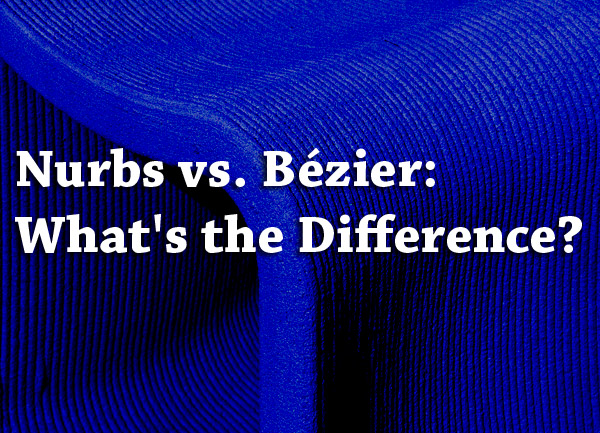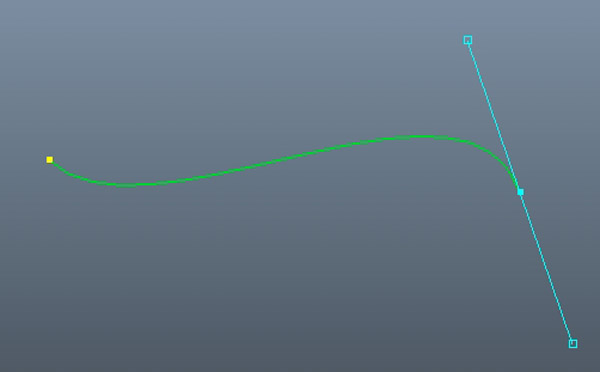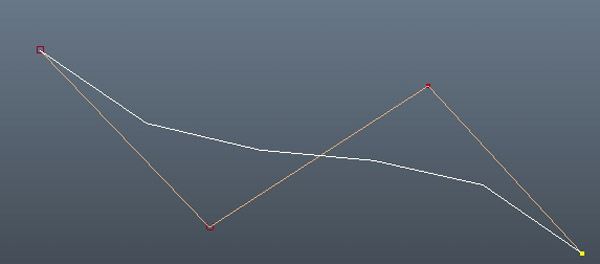# Nurbs vs. Bezier: What’s the Difference?

Nurbs, Beziers, B-splines, oh my!  If computer graphics curve and surface lingo has you confused at first, you are not alone. Hint: There is a connection to automobiles.  I have always wondered what reaction I would get if i had car vanity plates with one of these terms on them.After reading this article, you will not only know the difference, you will be able to proudly explain what the terms mean.

Here are the differences between NURBS and Bezier.  NURBS curves and surfaces are generalizations of Bezier curves and surfaces.  A Bezier curve is a single segment whereas NURBS curves are multisegment.  Bezier curves have two end points and two control points for the tangent vectors whereas NURBS curves are defined by four control points.

This is the short answer.  Now lets define what the different terms mean and how they all relate.

## Bezier

The Bezier curve is actually named after Pierre Bezier who used it to design the bodies of Renault vehicles.  They are common in computer graphics in programs such as Adobe Illustrator when you use vector graphics and can be scaled indefinitely.  In image editing programs they are called “paths” and are smooth.   Bezier curves are used in animation to outline movement, for example in easing.  They are also used for fonts.The Bezier curve has 2 end points, one at each end of the curve, and possibly also control points in between those 2, which do not lie on the curve but give the curve its actual curvature.

Bezier curves have all their control points averaged where the weights add up to one.   This means that such a curve always lies within the convex hull of its control points.  Bezier curves are non rational splines.   Bezier curves use handles for editing, not control vertices which are offset from the curve.  Bezier curves can have round curves as well as sharp corners which makes them useful for graphic design. They cant represent a circle exactly whereas rational splines such as NURBS can.

Interestingly, you can import Illustrator curves into 3d packages like Maya and then use them to create 3D surfaces.  Some 3d programs have Beziers like 3Ds Max, while others  do not.

Here is a mathematical explanation of Beziers.

## NURBS

NURBS stands for Non-Uniform Rational B-Splines.  NURBS is a mathematical model for the creation of both curves and surfaces.  These curves are  based on organic mathematics  and are therefore good for organic models.NURBS  are “rational” as the name suggests, which means they have non- homogenous weighted control points. Other types of non-rational or simple B-splines have an equal weight of 1 at each control point.The higher the weight of a control vertex, the more the curve segment is pulled toward that control vertex.  Points on NURBS curves are computed by taking a weighted sum of several control points.

NURBS surfaces are actually created when isoparms, the underlying curves, are connected to form spans (also called spanning the curves).  More spans means more detail. Isoparms can be divided into spans (isoparms that run horizontally in a surface) and sections (isoparms that run vertically in a surface).  Isoparms are surface curves and they are always on the surface itself.

A NURBS surface can be smoothly manipulated by moving around the control vertices which can also be animated.  NURBS surfaces can be made by various methods, such as the surface connecting 4 separate curves, a curve being extruded along another curve, a curve being revolved around and axis, etc.

Eventually, an entire NURBS model is formed by a series of NURBS surfaces, called patches, sort of like when a large blanket is sewn together from rectangular pieces created by individuals.  This is often a complicated process as positional, tangential and curvature continuity must all be maintained between patches.

NURBS models are not used for realtime applications such as games; they are used mainly in animation where the output is rendered and then displayed at a later time.   This type of modeling is best suited for organic shapes, as it is very good at creating lines and curved shapes.  NURBS are good because you can get a much smoother surface than a polygon model while using few control vertices, and therefore a lighter model that is better at deforming.

Keep in mind that at render time, NURBS surfaces are all tesselated, or converted into polygons.

Here is a mathematical explanation of NURBS.

## B-splines

A B-spline also called a basis-spline is a smooth curve that follows a control polygon made of control points.  It is a generalization of a Bezier curve.

There are various B spline types such as a Cardinal B-spline, with a constant separation between knots.  A NURBS curve or non-rational B spline is a B-spline where the control points all have a weight.  The weighting of the control points makes the curve rational.  In NURBS, a point is computed by calculating a weighted sum of several control points.

Here is a mathematical explanation of B-splines.

In the video below, you can watch Bezier and NURBS curves visually compared which should make it easier to understand:

I hope this article made NURBS, Beziers, and B-splines a little less of a mystery.  Take a look at other 3d graphics related articles on the site.

Doc J

Dr. J has worked in biology all his life and holds several advanced degrees and certificates in biology, anatomy, scientific illustration, 3d animation and motion graphics. He has always had an interest in teaching others the wonders of biology using the latest advances in graphics, including visualization, digital microscopy, animation, illustration, VR and interactive media.

### Recent Posts

All content, including text, graphics, images and information, contained on or available through this web site is for general information purposes only.